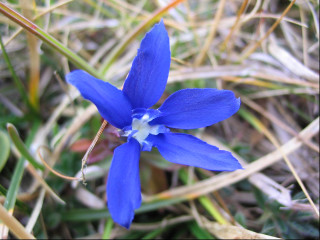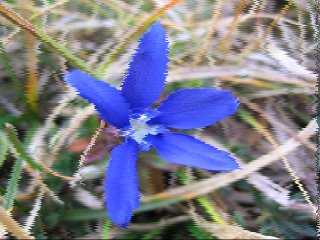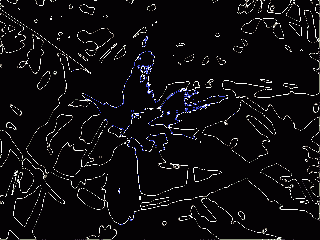(Last updated: 30/05/15)

## Samples gallery

Here's a selection of DogLua scripts in action! The following gallery show the resulting effect of the script applied to the reference image, along with the script source, ready to be copy & pasted. Credit is given were due; if you have coded some interesting script, feel free to send it (see the contacts page) for addition.
Be sure to check also the galleries at gluas site and The Best 3D.

N.B. Due to the size of the screenshots, the page may take a while to load.

Reference ImageFlipMix

Mix the source with a specular copy of it.for y = 0, height - 1 do for x = 0, width - 1 do r1, g1, b1 = get_rgb(x, y) r2, g2, b2 = get_rgb(width - 1 - x, y) r = (r1 + r2) / 2 g = (g1 + g2) / 2 b = (b1 + b2) / 2 set_rgb(x, y, r, g, b) end progress(y / height) end

Color Thresholdlevel = 0.5 function threshold(val) if val < level then return 0 else return 1 end end for y = 0, height - 1 do for x = 0, width - 1 do r, g, b = get_rgb(x, y) r = threshold(r) g = threshold(g) b = threshold(b) set_rgb(x, y, r, g, b) end progress(y / height) end

Amiga's HAM (by me & Dan Ritchie)

This filter try to mimic the effect of the special Amiga's graphic mode Hold-And-Modify, or HAM. I started quantizing the RGB components in the 4bit range, but Dan come up with the better idea of starting with a dithered conversion to 12bit color before appling the filter.-- First dither the image using an -- existing plugin Dog_ShellExe("12_bit_dither_pf.exe") -- and reload the buffer Dog_GetBuffer() -- Determine the greater value of 3 function max_p(x1, x2, x3) local v if x1 > x2 then if x1 > x3 then return 1 else return 3 end else if x2 > x3 then return 2 else return 3 end end end -- Works only in the selected area for y = bound_y0, bound_y1 do lr = 0 lg = 0 lb = 0 for x = bound_x0, bound_x1 do r, g, b = get_rgb(x, y) -- Compute difference with last pixel dr = math.abs(r - lr) dg = math.abs(g - lg) db = math.abs(b - lb) -- Change the most different component v = max_p(dr, dg, db) if v == 1 then lr = r elseif v == 2 then lg = g else lb = b end set_rgb(x, y, lr, lg, lb) end progress((y - bound_y0) / (bound_y1-bound_y0)) end

Slider

A multi-pass filter. On every round, some random selected scanlines are left shifted.function slide(y) back_r, back_g, back_g = get_rgb(0,y) for x = 0, bound_x1 - 1 , 1 do r, g, b = get_rgb(x + 1, y) set_rgb(x, y, r, g, b) end set_rgb(width - 1, y, back_r, back_g, back_g) end rep = Dog_ValueBox("Slider", "Repeat:", 1, 50, 5) prob = Dog_ValueBox("Slider", "Probability:", 1, 20, 5) counter = 0 maxcounter = rep * (bound_y1 - bound_y0) for t = 1, rep, 1 do for y = bound_y0, bound_y1, 1 do c = math.random(prob) if c == 1 then slide(y) end counter = counter + 1 progress(counter / maxcounter) end flush() Dog_Refresh() end

Life

An implementation of the famous Conway's game of Life for DogLua.-- times to iterate the process maxgen = 50 function cell(x, y) -- check a cell state if get_value(x, y) > 0.5 then return 1 else return 0 end end function living(x, y) -- check if a cell will live -- depending on his 8 neighbors v = cell(x - 1, y - 1) + cell(x, y - 1) + cell(x + 1, y - 1) + cell(x - 1, y) + cell(x + 1, y) + cell(x - 1, y + 1) + cell(x, y + 1) + cell(x + 1, y + 1) if v < 2 then -- will die for loneliness return -1 elseif v > 3 then -- will die for crowding return -1 elseif v == 3 then -- new cell come to life! return 1 else -- nothing change return 0 end end totcycles = maxgen * (bound_y1 - bound_y0) cycle = 0 for g = 1, maxgen do for y = bound_y0, bound_y1 do for x = bound_x0, bound_x1 do v = living(x, y) if v == 1 then set_value(x, y, 1) elseif v == -1 then set_value(x, y, 0) end end cycle = cycle + 1 progress(cycle / totcycles) end flush() Dog_Refresh() end